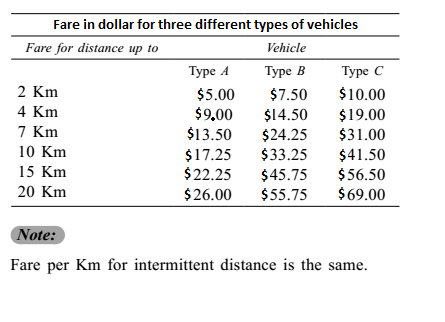## Introduction to Data Interpretation

#### Data Interpretation

Direction: Study the following table carefully and answer the questions given below it.1. Shiv Kumar has to travel a distance of 15 Km in all. He decides to travel equal distance by each of the three types of vehicles. How much money is to be spent as fare?

1. ∴ Total fare = Fare of A + Fare of B + Fare of C

##### Correct Option: D

Distance to be travelled by each type of vehicle

 = 15 = 5 km 3

Since, to travel 5 Km by vehicle A, he will pay \$ 9 for 4 Km and for the next 1 Km he will have to pay
 \$ (13.5 - 9.00 ) x 1 ( 7 - 4 )

Similarly, for other cases.
 Fare by A = \$ 9 + (13.50 - 9 ) = 9 + 1.50 = \$ 10.50 ( 7 - 4 )

 Fare by B = 14.50 + ( 24.25 - 14.50 ) = 14.50 + 3.25 = \$ 17.75 ( 7 - 4 )

 Fare by C = 19 + ( 31 - 19 ) = 19 + 4 = \$ 23 ( 7 - 4 )

∴ Total fare = Fare of A + Fare of B + Fare of C
Total fare = 10.50 + 17.75 + 23 = \$ 51.25.

1. Rita hired a Type B vehicle for travelling a distance of 18 Km. After travelling 5 Km, she changed the vehicle to type A. Again, after travelling 9 Km by Type A vehicle, she changed the vehicle to Type C and completed her journey. How much money did she spend did in all?

1. ∴ Total fare = Fare by A for 8 Km + Fare by B for 5 km + Fare by C for 5 Km

##### Correct Option: E

Fare by B for 5 km = 14.50 + 3.25 = \$ 17.75

 Fare by A for 8 Km = 13.50 + 17.25 - 13.50 = \$ 14.75 3

 Fare by C for 5 Km = 19 + 31 - 19 = \$ 23 3

∴ Total fare = Fare by A for 8 Km + Fare by B for 5 km + Fare by C for 5 Km
Total fare = 17.75 + 14.75 + 23 = 55.50.

1. Ajit Singh wants to travel a distance of 15 Km. He starts his journey by Type A vehicle. After travelling 6 Km, he changes the vehicle to Type B for the remaining distance. How much money will he be spending in all?

1. ∴ Total fare = Fare by A + Fare by B

##### Correct Option: A

 Fare by A = \$ 9 + 4.50 = \$ 12 3 x 2

 Fare by B = \$ 24.25 + 33.25 - 24 = \$ 30.25 3 x 2

∴ Total fare = Fare by A + Fare by B
∴ Total fare = 30.25 + 12 = \$ 42.25.

1. Fare for 14th Km by Type C vehicle is equal to the fare for which of the following?

1.  Fare for 14th Km by C = 56.50 - 41.50 = \$ 3 15 - 10

##### Correct Option: B

 Fare for 14th Km by C = 56.50 - 41.50 = \$ 3 15 - 10

 Fare for 9th Km by B = 33.25 - 24.25 = \$ 3 10 - 7

1. Mr X wants to travel a distance of 8 Km by Type A vehicle. How much more money will be required to be spent if he decides to travel by Type B vehicle instead of Type A?

1. ∴ Difference = Fare by B − Fare by A

##### Correct Option: B

 Fare, for 8 Km by A = 13.50 + 17.25 - 13.50 = 13.50 + 3.75 = \$ 14.75 10 - 7 3

 Fare by B = 24.25 + 33.25 - 24.25 = \$ 27.25 3

∴ Difference = Fare by B − Fare by A
Difference = 27.25 − 14.75 = \$ 12.50.